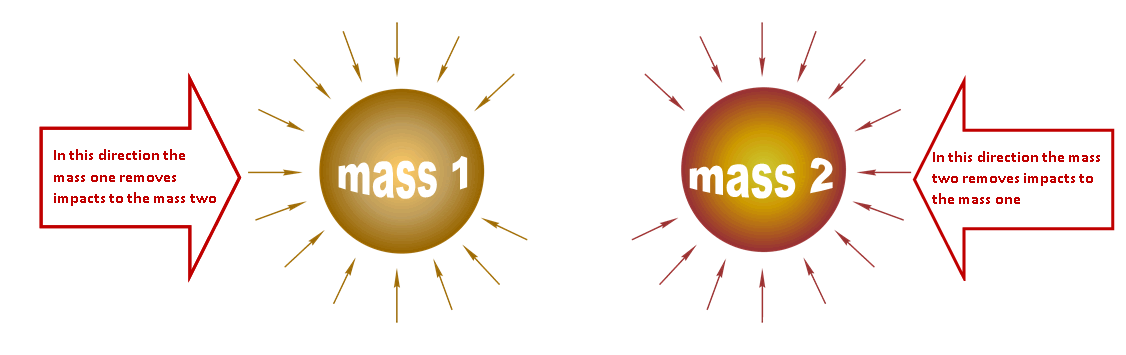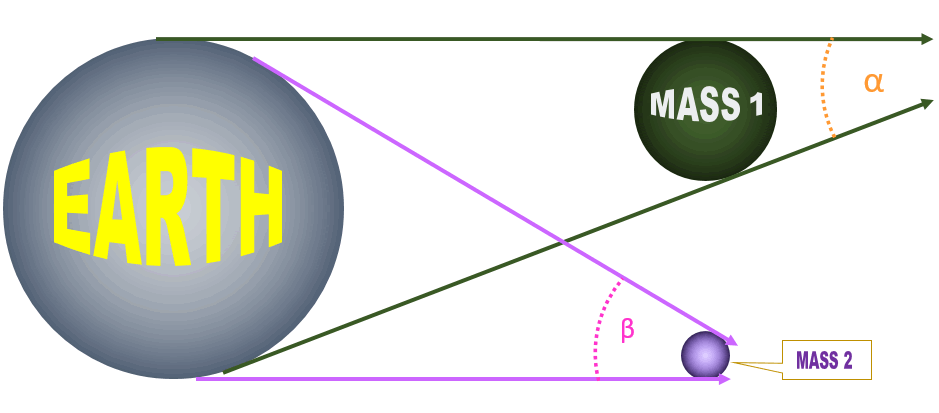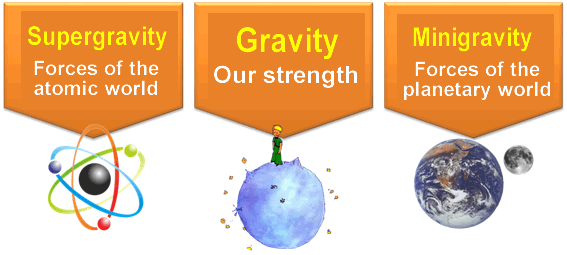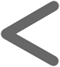Imagine a neutral particle so small, so small that it crosses the known universe in a sigh

félix martín gómez

#### NEUTRAL ACTION

Trillions and trillions of neutral particles are continually traversing us and it only impacts one particle of those trillions of particles.
Matter is continuously traversed by trillions of neutral particles.

#### Weak atomic force

Observe only the neutral particles that impact, millions, against matter, against us.

As the matter is hollow, only one particle impacts, of the trillions of particles that cross us, But still there are many particles that impact, producing a neuter force of compression millions of times more powerful than the forces known by us and it is known asweak atomic force.

The particles impact against the basic parts of matter, atoms.
These particles disappear as neutral particles and are transformed into electrical particles, we will see in the next chapter as "reacción".

Each atom of matter is fixed in space by this enormous neuter force of compression, atomic force of neutral character.

Atoms, as a basic part of matter, are a small obstacle to the movement of these neutral particles, the atoms behaving like wells or sinks of a part of that movement that fills the space.

this neuter atomic force so powerful we do not notice it because we are formed by molecules, the molecules are formed by atomss, But the molecular forces are much weaker and it is these molecular forces, much weaker, that affect us.
It is the molecular forces that interact, bond atoms, and form matter.
Between atom and atom there is a huge distance that us protects, minimizes, atomic force.Figure 3 The matter receives millions of impacts, from these neuter miniparticles, from the all directions. These impacts are received by the basic components of matter, the atoms, and produce a neuter force millions of times more powerful than the neuter forces we know. It is an atomic force known as WEAK ATOMIC FORCE. The masses are formed by many atoms. There is an immense distance between atom and atom –practically hollow mass- And the enormous distance separating one atom from the other atom allows this powerful neutral atomic force not to manifest itself at the global level of mass.

#### Inertial point zero

The impacts of neutral particles are the equal in all spatial directions, So the atom is in its inertial point zero.
Equal impacts from all directions.
If they do not receive the same impacts from all directions, the atom will move toward the direction where it receives the less impacts.
The simple law of action and reaction, the side where there are more impacts shifts the atom to where it receives less impacts.
Consequently:

All atoms are, or tend to be, at its zero inertial point.

As the masses are made up of millions of atoms united in a mega structure, we can also say:

All the masses are, or tend to be,En su punto cero inercial

A mass which is at its zero inertial point is a mass at rest, Although all mass may be in relative motion with respect to other masses.

#### Inertia

When two masses impact, interact or collide, what they do is to adapt their relative rest or zero inertial points.

From the outside you can only act over a few atoms of the mass, on the atoms of the outer layers of the mass.

The inertia is born in that tension that is generated in the action on a few atoms that come in contact and the reaction of the millions of inner atoms, that do not directly impact and will follow in its zero inertial point.

Action on the few outer atoms of matter, reaction of the millions of atoms inside the matter.

Inertia is the tension generated between the action on a few and the reaction of many.
If the action is abrupt breaks the molecular bond, it breaks the matter.Figure 4 Matter is hollow, there is an immense distance between atom and atom. You can only act on a few atoms, the outer ones, the atoms of the outermost layer of matter. Each atom is fixed in space by weak the atomic force, forces millions of times more powerful than the molecular forces that bind atoms. INERCIA IS: Action of a few atoms, Atoms joined by molecular forces - weak forces -. Reaction of many atoms fixed to space by weak the atomic force, millions of times more powerful than molecular forces. If the action, impact, is abrupt and gives no time to compensate for the inertial point of the different layers of atoms, breaks the molecular bond, breaks matter.

#### The gravity

When there are two nearby masses, the impacts of these neutral particles on the masses can not be equal in the three directions of space.
The particles that have to pass through the other mass have to pass through it and take away impacts in that direction.
One dough makes screen to the other dough.

The particles that impact on the first mass will no longer do so on the next mass.
The masses will receive fewer impacts in the direction of the other masses, by so that the masses will tend to come together.

Fact that does not happen, we do not notice that a mass, at a distance, is affected by another mass.

This apparent incongruity is not if we think that matter is practically hollow.
At atomic level there is a huge distance between one atom and other atom so the screen effect between masses is very weak, even in large masses.

We ponder that in small masses it is not detectable, even if it is a huge mountain.

What if the mass that makes of shield is a huge mass?
For example, with 12,000 kilometers in diameter?
For example, the planet Earth?

Do we notice this screen action that produces the mass of our planet?

Well, it shows!

We notice it because the particles that have to traverse Earth are a few less.
We notice that on the opposite side of the Earth we receive more impacts and we can see in what we are stuck to the planet.
This effect we call GRAVITY.Figure 5 Two neighboring masses are made screen, with respect to these neutral miniparticles, reason why an effect of attraction between masses takes place. The force of attraction is so weak that it is not appreciated, even in masses as large as a mountain. On planet Earth, this screen effect allows the masses to stick to their surface and is known as GRAVITY. The screen effect is proportional to the mass, and the value it has on our planet is 1g or a terrestrial gravity.

That is gravity, a screen effect between masses with respect to the neutral particles.

The imagination of these particles, which traverse the universe in a sigh, converts la force of gravity, independent and unknown force, in an effect that a mass produces in the other masses.

(Galileo lamented the allegations that put him on what was said by Aristotle and said that "if Aristotle could hear me he would give me the reason". I too would now like to be able to present this to the seeker Grand Unified Theory, to Einstein; maybe he would give me the reason, at least he would like this way of seeing gravity.)

NOTE I For not to have to repeat:

"Imaginary very small and very fast neutral particles, millions of millions, moving equally in all directions of space and crossing the known universe in a sigh"
And after seeing that they give meaning to inertia and gravity, From now on we will refer to them as "etherinos".

#### Supergravity

The weak atomic force is:

• Millions of impacts of the etherino on the atoms.

Gravity is:

• The lack of a few impacts of the etherino.

Then weak atomic force and gravity are of equal nature, Being the atomic weak force millions of times more powerful than the gravity reason why SUPERGRAVITY is the atomic weak force.

NOTE II It was said that any mass is or tends to be at its zero inertial point, all equal impacts in the three spatial directions.
Now we see that the masses that are in a gravitational field have the unbalanced impacts, there are more impacts on one side than on the other, so that the masses in a gravitational field are affected to a uniform acceleration.
The gravity effect produces in the masses a uniformly accelerated movement, which tends to bring these masses towards their zero inertial point.

#### Minigravity

In laboratories it is believed that there is another basic force, it would be the fifth basic force, although it is not very sure of it.

Fifth force, two equal masses, but of different density have different gravity, dropping, in the void, a pen and an iron ball, first falls the pen.

This force is not made known because it is very weak and does not find justification to its existence.

Continuing with this study, Assuming the existence of the ether, we can see that the shadow angle (figure 6), With regard to the etherinos, That makes the Earth, on two masses of equal weight, but different density, have different angles.
It makes more shadow on the mass with less density, reason why the gravity is greater in the mass number one than in the mass number two (the pen falls first than the iron ball).Figure 6 The angle of shadow, with respect to the etherino, that makes the Earth on two masses of equal weight, but different density, have different angles. The Earth makes more shadow on the mass with less density, that causes that the gravity is slightly greater in mass number one than in mass number two.

The fifth force is a force neutral similar to gravity, but millions of times weaker, That's why we're going to call her MINIGRAVITY.
Es tan débil la minigravedad que tal vez no logremos diseñar un experimento fiable.

To avoid measurement errors in the fall of bodies, we can make a device (figure 7) than marks the difference of attraction not in fall but in suspension, and search the difference of attraction on other celestial bodies as they are the Moon or the Sun.Figure 7 DETECTOR OF THE FIFTH FORCE. Two masses of equal weight, but very different densities, when suspended in equilibrium, will rotate freely and will be oriented in the direction of any field or force acting differently on masses with different density. It will only rotate in the presence of fields or forces acting horizontally; The forces in the vertical direction are balanced; the forces acting vertically are balanced. The less dense mass, the aluminum, will be oriented towards the Moon or the Sun when one is in the sunset and the other in the zenith. The gravities of the Moon or the Sun will act differently on the masses with different density.

We already saw the problems in the detection of gravity, For being gravity a weak force, to detect it we had to resort to a great mass, how to it be the earth.

So we are going to look for a great mass that can serve ascompass gravitational or detector fifth force .
Let's search for a large mass with different densities in your hemispheres.
Great masses with the hemispheres with different densities we have them in some moons of our planetary system, as is the case of our Moon, which have different density in their halves.
The behavior as a gravitational compass or detector of the fifth force will be the: Have the face with less density oriented towards the planet to which it orbits,That is to say that it will always show the same face to the planet to which it orbits.

The fact that the Moon always shows the same face is due to the fifth force, which manifests itself as "a gravitational compass", Neutral force very, very weak manifested at planetary level and that we have baptized like very small gravity.

#### Forces of the "NEUTRAL ACTION"

Here ends the part that we denominate like action of the etherinos ones on the masses or on the matter.

We can sum it up as:

Supergravity, Very, very strong neutral force acting at atomic level.

Gravity, Weak force our strength.

Minigravity, Very, very weak force acting at planetary level.Knowledge Reaction